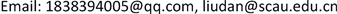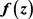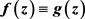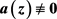﻿ 整函数差分唯一性 Uniqueness of Difference about Entire Functions

Pure Mathematics
Vol. 09  No. 03 ( 2019 ), Article ID: 30322 , 7 pages
10.12677/PM.2019.93049

Uniqueness of Difference about Entire Functions

Xiaohuang Huang, Dan Liu

Institute of Applied Mathematics, South China Agricultural University, Guangzhou GuangdongReceived: Apr. 26th, 2019; accepted: May 6th, 2019; published: May 21st, 2019ABSTRACT

In this paper, we investigate the uniqueness of difference operators about entire function, and prove: letbe an entire function of finite order, k be some positive integers, let $a\left(z\right)$ be a small function of $f\left(z\right)$ , and let $g\left(z\right)={m}_{1}\left(z\right)f\left(z+{c}_{1}\right)+\cdots +{m}_{k}\left(z\right)f\left(z+{c}_{k}\right)$ be the difference polynomial of $f\left(z\right)$ , where ${m}_{i}\left(z\right)\left(i=1,2,\cdots ,k\right)$ are the small functions of $f\left(z\right)$ , and ${c}_{i}\left(i=1,2,\cdots ,k\right)$ are some finite distinct values. If $f\left(z\right)$ and $g\left(z\right)$ share 0 CM, and share $a\left(z\right)$ IM, then.

Keywords:Entire Function, Shared Small Function, Difference Polynomials$g\left(z\right)={m}_{1}\left(z\right)f\left(z+{c}_{1}\right)+\cdots +{m}_{k}\left(z\right)f\left(z+{c}_{k}\right)$

$f\left(z\right)$ 的差分多项式，其中 ${m}_{i}\left(z\right)\left(i=1,2,\cdots ,k\right)$ 为f的整小函数， ${c}_{i}\left(i=1,2,\cdots ,k\right)$ k个判别的有穷复数。又设$f\left(z\right)$ 的一个小函数，若 $f\left(z\right)$$g\left(z\right)$ 分担0，IM分担 $a\left(z\right)$ ，则 $f\left(z\right)\equiv g\left(z\right)$Copyright © 2019 by author(s) and Hans Publishers Inc.1. 引言

$\begin{array}{l}g\left(z\right)={m}_{1}\left(z\right)f\left(z+{c}_{1}\right)+{m}_{2}\left(z\right)f\left(z+{c}_{2}\right)\\ \text{\hspace{0.17em}}\text{\hspace{0.17em}}\text{\hspace{0.17em}}\text{\hspace{0.17em}}\text{\hspace{0.17em}}\text{\hspace{0.17em}}\text{\hspace{0.17em}}\text{\hspace{0.17em}}\text{\hspace{0.17em}}\text{\hspace{0.17em}}+\cdots +{m}_{k}\left(z\right)f\left(z+{c}_{k}\right)\end{array}$

$f\left(z\right)$ 的差分多项式。最近，许多人做了关于复差分唯一性的问题。2014年，Liu-Fang  证明了：

2017年，Li-Duan-Chen  证明了：

$g\left(z\right)={m}_{1}\left(z\right)f\left(z+{c}_{1}\right)+{m}_{2}\left(z\right)f\left(z+{c}_{2}\right)+\cdots +{m}_{k}\left(z\right)f\left(z+{c}_{k}\right)$

$f\left(z\right)$ 的差分多项式，其中 ${m}_{i}\left(z\right)\left(i=1,2,\cdots ,k\right)$ 为f的整小函数， ${c}_{i}\left(i=1,2,\cdots ,k\right)$ k个判别的有穷复数。又设 $a\left(z\right)\overline{)\equiv }0$$f\left(z\right)$ 的一个小函数，若 $f\left(z\right)$$g\left(z\right)$ 分担0，IM分担 $a\left(z\right)$ ，则 $f\left(z\right)\equiv g\left(z\right)$

2. 几个引理

$m\left(r,\frac{f\left(z+c\right)}{f\left(z\right)}\right)=S\left(r,f\right)$ ,

$m\left(r,\frac{P\left(f\right){f}^{\prime }}{\left(f-{b}_{1}\right)\left(f-{b}_{2}\right)\cdots \left(f-{b}_{q}\right)}\right)=S\left(r,f\right)$ .

$\begin{array}{l}N\left(r,{f}_{1}{f}_{2}\right)-N\left(r,\frac{1}{{f}_{1}{f}_{2}}\right)\\ =N\left(r,{f}_{1}\right)+N\left(r,{f}_{2}\right)-N\left(r,\frac{1}{{f}_{1}}\right)-N\left(r,\frac{1}{{f}_{2}}\right)\end{array}$ ,

$m\left(r,P\left(z,f\right)\right)=S\left(r,f\right)$ .

$g\left(z\right)={m}_{1}\left(z\right)f\left(z+{c}_{1}\right)+{m}_{2}\left(z\right)f\left(z+{c}_{2}\right)+\cdots +{m}_{k}\left(z\right)f\left(z+{c}_{k}\right)$

$f\left(z\right)$ 的差分多项式，其中 ${m}_{i}\left(z\right)\left(i=1,2,\cdots ,k\right)$$f\left(z\right)$ 的整小函数， ${c}_{i}\left(i=1,2,\cdots ,k\right)$ k个判别的有穷复数。则

i) $L\left(f\right)=|\begin{array}{cc}f& a\\ {f}^{\prime }& {a}^{\prime }\end{array}|\overline{)\equiv }0$$L\left(g\right)=|\begin{array}{cc}g& a\\ {g}^{\prime }& {a}^{\prime }\end{array}|\overline{)\equiv }0$

ii) $m\left(r,\frac{L\left(f\right)}{f-{d}_{j}}\right)=S\left(r,f\right)$ ，与 $m\left(r,\frac{L\left(f\right)f}{\left(f-{d}_{1}\right)\left(f-{d}_{2}\right)\cdots \left(f-{d}_{m}\right)}\right)=S\left(r,f\right)$ ，其中 $2\le m\le q$

i) 只证 $L\left(f\right)\overline{)\equiv }0$ 。若不然，有 $L\left(f\right)\equiv 0$ ，即 ${a}^{\prime }f-a{f}^{\prime }\equiv 0$ 。上式两边积分可得 $f\equiv Da$ ，其中D为非零常数。由特征函数关系明显有 $T\left(r,f\right)=T\left(r,a\right)=S\left(r,f\right)$ ，这显然不可能。故 $L\left(f\right)\overline{)\equiv }0$ 。同理可证 $L\left(g\right)\overline{)\equiv }0$

ii) 显然有 $m\left(r,\frac{L\left(f\right)}{f-d}\right)=m\left(r,\frac{{a}^{\prime }\left(f-d\right)-\left({f}^{\prime }-{d}^{\prime }\right)}{f-d}\right)\le S\left(r,f\right)$ ，故 $m\left(r,\frac{L\left(f\right)}{f-{d}_{j}}\right)=S\left(r,f\right)$ 。因为 $\frac{L\left(f\right)f}{\left(f-{d}_{1}\right)\left(f-{d}_{2}\right)\cdots \left(f-{d}_{m}\right)}=\underset{i=1}{\overset{m}{\sum }}\frac{L\left(f\right)}{f-{d}_{i}}$ ，从而根据上式可证得 $m\left(r,\frac{L\left(f\right)f}{\left(f-{d}_{1}\right)\left(f-{d}_{2}\right)\cdots \left(f-{d}_{m}\right)}\right)=S\left(r,f\right)$

$T\left(r,f\right)\le \stackrel{¯}{N}\left(r,\frac{1}{f-a}\right)+\stackrel{¯}{N}\left(r,\frac{1}{f-b}\right)+\stackrel{¯}{N}\left(r,\frac{1}{f-c}\right)+S\left(r,f\right)$ .

3. 定理1的证明

$\begin{array}{c}T\left(r,f\right)\le \stackrel{¯}{N}\left(r,\frac{1}{f}\right)+\stackrel{¯}{N}\left(r,\frac{1}{f-a}\right)+S\left(r,f\right)\\ =\stackrel{¯}{N}\left(r,\frac{1}{g}\right)+\stackrel{¯}{N}\left(r,\frac{1}{g-a}\right)+S\left(r,f\right)\\ \le N\left(r,\frac{1}{f-g}\right)+S\left(r,f\right)\le T\left(r,f-g\right)+S\left(r,f\right)\\ \le m\left(r,f-g\right)+S\left(r,f\right)\le m\left(r,f\right)+m\left(r,1-\frac{g}{f}\right)+S\left(r,f\right)\\ \le T\left(r,f\right)+S\left(r,f\right)\end{array}$ ,

$T\left(r,f\right)=\stackrel{¯}{N}\left(r,\frac{1}{f}\right)+\stackrel{¯}{N}\left(r,\frac{1}{f-a}\right)+S\left(r,f\right)$(1)

$\phi =\frac{L\left(f\right)\left(f-g\right)}{f\left(f-a\right)}$(2)

$\varphi =\frac{L\left(g\right)\left(f-g\right)}{g\left(g-a\right)}$ , (3)

$\begin{array}{c}T\left(r,\phi \right)=m\left(r,\phi \right)+N\left(r,\phi \right)\\ =m\left(r,\frac{L\left(f\right)\left(f-g\right)}{f\left(f-a\right)}\right)+S\left(r,f\right)\\ \le m\left(r,\frac{L\left(f\right)}{f\left(f-a\right)}\right)+m\left(r,1-\frac{g}{f}\right)+S\left(r,f\right)\\ =S\left(r,f\right)\end{array}$ , (4)

$T\left(r,\phi \right)=S\left(r,f\right)$ 。设 $d=a-ja\left(j\ne 0,1\right)$ ，根据引理5有

$\begin{array}{c}m\left(r,\frac{1}{f}\right)=m\left(r,\frac{1}{a\phi }\left(\frac{L\left(f\right)}{f}-\frac{L\left(f\right)}{f-a}\right)\left(1-\frac{g}{f}\right)\right)\\ \le m\left(r,1-\frac{g}{f}\right)+S\left(r,f\right)\\ =S\left(r,f\right)\end{array}$ , (5)

$\begin{array}{c}m\left(r,\frac{1}{f-d}\right)=m\left(r,\frac{L\left(f\right)\left(f-g\right)}{\phi \left(f-a\right)\left(f-d\right)f}\right)\\ \le m\left(r,1-\frac{g}{f}\right)+m\left(r,\frac{L\left(f\right)f}{f\left(f-a\right)\left(f-d\right)}\right)+S\left(r,f\right)\\ =S\left(r,f\right)\end{array}$ . (6)

$\frac{g}{f}=\frac{{e}^{\partial }}{H}$ , (7)

$T\left(r,g\right)=T\left(r,{e}^{\alpha }f\right)+S\left(r,f\right)=T\left(r,f\right)+S\left(r,f\right)$ . (8)

$\begin{array}{c}2T\left(r,f\right)\le 2T\left(r,g\right)+S\left(r,f\right)\\ \le \stackrel{¯}{N}\left(r,\frac{1}{g}\right)+\stackrel{¯}{N}\left(r,\frac{1}{g-a}\right)+\stackrel{¯}{N}\left(r,\frac{1}{g-d}\right)+S\left(r,f\right)\\ \le \stackrel{¯}{N}\left(r,\frac{1}{g}\right)+\stackrel{¯}{N}\left(r,\frac{1}{f-a}\right)+T\left(r,\frac{1}{g-d}\right)-m\left(r,\frac{1}{g-d}\right)+S\left(r,f\right)\\ \le T\left(r,f\right)+T\left(r,g\right)-m\left(r,\frac{1}{g-d}\right)+S\left(r,f\right)\\ \le 2T\left(r,f\right)-m\left(r,\frac{1}{g-d}\right)+S\left(r,f\right)\end{array}$ ,

$m\left(r,\frac{1}{g-d}\right)=S\left(r,f\right)$(9)

$\begin{array}{c}m\left(r,\frac{f-d}{g-d}\right)\le m\left(r,\frac{f}{g-d}\right)+m\left(r,\frac{d}{g-d}\right)\\ \le T\left(r,\frac{f}{g-d}\right)-N\left(r,\frac{f}{g-d}\right)+S\left(r,f\right)\\ \le m\left(r,\frac{g-d}{f}\right)+N\left(r,\frac{g-d}{f}\right)-N\left(r,\frac{f}{g-d}\right)+S\left(r,f\right)\\ \le N\left(r,\frac{1}{f}\right)-N\left(r,\frac{1}{g-d}\right)+S\left(r,f\right)\\ =T\left(r,\frac{1}{f}\right)-T\left(r,\frac{1}{g-d}\right)+S\left(r,f\right)\\ =T\left(r,f\right)-T\left(r,g\right)+S\left(r,f\right)\end{array}$ .

(3)又可写成 $\varphi =\left[\frac{a-d}{a}\frac{L\left(g\right)}{g-a}+\frac{d}{a}\frac{L\left(g\right)}{g}\right]\left[\frac{f-d}{g-d}-1\right]$ ，则由(10)可得到

$T\left(r,\varphi \right)=m\left(r,\varphi \right)+N\left(r,\varphi \right)=m\left(r,\varphi \right)+S\left(r,f\right)=S\left(r,f\right)$ . (11)

${H}_{n,m}=n\phi -m\varphi$ ，其中 $m,n$ 为正整数。接下来我们分为以下几种情形讨论。

$n\left(\frac{{f}^{\prime }}{f}-\frac{{f}^{\prime }-{a}^{\prime }}{f-a}\right)\equiv m\left(\frac{{g}^{\prime }}{g}-\frac{{g}^{\prime }-{a}^{\prime }}{g-a}\right)$ ,

$nT\left(r,f\right)=mT\left(r,g\right)+S\left(r,f\right)$ ,

$\frac{f}{f-a}\equiv B\frac{g}{g-a}$ , (12)

$f\left[\left(B-1\right)g+a\right]\equiv Bag$ . (13)

$m\left(r,\left(B-1\right)g+a\right)=S\left(r,f\right)$ , (14)

$m\left(r,g\right)=S\left(r,f\right)$ 。这可推出 $T\left(r,f\right)=T\left(r,g\right)+S\left(r,f\right)=m\left(r,g\right)+S\left(r,f\right)=S\left(r,f\right)$ ，显然矛盾。

$\begin{array}{l}{\stackrel{¯}{N}}_{\left(m,n\right)}\left(r,\frac{1}{f}\right)+{\stackrel{¯}{N}}_{\left(m,n\right)}\left(r,\frac{1}{f-a}\right)\\ \le \stackrel{¯}{N}\left(r,\frac{1}{n\phi -m\varphi }\right)+S\left(r,f\right)\le T\left(r,n\phi -m\varphi \right)+S\left(r,f\right)\\ \le T\left(r,\phi \right)+T\left(r,\varphi \right)+S\left(r,f\right)=S\left(r,f\right)\end{array}$ . (15)

$\begin{array}{c}T\left(r,f\right)=\stackrel{¯}{N}\left(r,\frac{1}{f}\right)+\stackrel{¯}{N}\left(r,\frac{1}{f-a}\right)+S\left(r,f\right)\\ \le {\stackrel{¯}{N}}_{1\right)}\left(r,\frac{1}{f}\right)+\underset{k=2}{\overset{4}{\sum }}{\stackrel{¯}{N}}_{k}\left(r,\frac{1}{f}\right)+{\stackrel{¯}{N}}_{\left(5}\left(r,\frac{1}{f}\right)+{\stackrel{¯}{N}}_{1\right)}\left(r,\frac{1}{f-a}\right)\\ \text{\hspace{0.17em}}\text{\hspace{0.17em}}+\underset{k=2}{\overset{4}{\sum }}{\stackrel{¯}{N}}_{k}\left(r,\frac{1}{f-a}\right)+{\stackrel{¯}{N}}_{\left(5}\left(r,\frac{1}{f-a}\right)+S\left(r,f\right)\\ \le {\stackrel{¯}{N}}_{\left(1,1\right)}\left(r,\frac{1}{f}\right)+\underset{k=2}{\overset{4}{\sum }}{\stackrel{¯}{N}}_{k}\left(r,\frac{1}{f}\right)+{\stackrel{¯}{N}}_{\left(5}\left(r,\frac{1}{f}\right)+\underset{m=1}{\overset{5}{\sum }}{\stackrel{¯}{N}}_{\left(1,m\right)}\left(r,\frac{1}{f-a}\right)\\ \text{\hspace{0.17em}}\text{\hspace{0.17em}}+\underset{k=2}{\overset{4}{\sum }}{\stackrel{¯}{N}}_{k}\left(r,\frac{1}{f-a}\right)+{\stackrel{¯}{N}}_{\left(5}\left(r,\frac{1}{f-a}\right)+S\left(r,f\right)\end{array}$

$\begin{array}{l}\le \frac{1}{5}\left[N\left(r,\frac{1}{f}\right)+N\left(r,\frac{1}{f-a}\right)\right]+\frac{1}{5}\left[N\left(r,\frac{1}{g}\right)+N\left(r,\frac{1}{g-a}\right)\right]+S\left(r,f\right)\\ \le \frac{2}{5}\left(T\left(r,f\right)+T\left(r,g\right)\right)+S\left(r,f\right)\\ =\frac{4}{5}T\left(r,f\right)+S\left(r,f\right)\end{array}$ (16)

$T\left(r,f\right)=S\left(r,f\right)$ 。矛盾。于是定理1得证。

Uniqueness of Difference about Entire Functions[J]. 理论数学, 2019, 09(03): 370-376. https://doi.org/10.12677/PM.2019.93049

1. 1. Yang, L. (1993) Value Distribution Theory. Springer-Verlag, Berlin.

2. 2. Hayman, W.K. (1964) Meromorphic Functions. Oxford University Press, London.

3. 3. Liu, D., Yang. D.G. and Fang, M.L. (2014) Unicity of Entire Functions Concerning Shifts and Dif-ference Operators. Abstract and Applied Analysis, 2014, Article ID: 380910.
https://doi.org/10.1155/2014/380910

4. 4. Li, S., Duan, M. and Chen, B.Q. (2017) Uniqueness of Entire Functions Sharing Two Values with Their Difference Operators. Advances in Difference Equations, Paper No. 390, 9 p.
https://doi.org/10.1186/s13662-017-1444-3

5. 5. Heittokangas. Korhonen, R., Laine, I. and Rieppo, J. (2011) Uniqueness of Meromorphic Functions Sharing Values with their Shifts. Complex Variables and Elliptic Equations, 56, 81-92.
https://doi.org/10.1080/17476930903394770

6. 6. Chiang, Y.M. and Feng, S.J. (2008) On the Nevanlinna Characteristic of f(z + η) and Difference Equations in the Complex Plane. The Ramanujan Journal, 16, 105-129.
https://doi.org/10.1007/s11139-007-9101-1

7. 7. Halburd, R.G. and Korhonen, R.J. (2006) Nevanlinna Theory for the Difference Operator. Annales Academiæ Scientiarum Fennicæ, 31, 463-478.

8. 8. Laine, I. and Yang. C.C. (2007) Clunie Theorems for Difference and q-Difference Polynomials. Journal of the London Mathematical Society, 76, 556-566.
https://doi.org/10.1112/jlms/jdm073